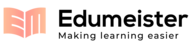## Lec6.pdf-Lecture 5 written notes (#4) 2012-01-25showing page 1-3 out of 3

##### Page 1
1/3
Lecture 5 written notes (#4)
2012-01-25 17:56:16
Lecture 5
• If our potential is infinite, our wavefunction must be zero there,
or else 'pay' an infinite energy to be there.
• If our potential isn't infinite, then the wavefunction can extend
into that zone at some energy 'price'.
• If the energy of our state, E, is greater than the barrier potential,
Uo, then the wavefunction can drift right over the barrier;
it will
have its kinetic energy reduced, though.
• If the energy of our state, E, is less than the barrier potential, Uo,
then we can't put the whole wavefunction over the barrier, but we
could put part of it into that zone...
THE DIFFERENCE with classical mechanics?
• in classical mechanics, the particle is localized, even to a single
point -- where it is in x tells you the whole information about what
the precise potential energy of the particle is. If that energy is
greater than Uo, the particle can ride over the potential barrier.
• in quantum mechanics, the particle is delocalized as a wave -- it
is not at a particular x, it is everywhere, and the potential energy
does not have the same meaning.
The whole wavefunction 'knows'
about the whole barrier, and the whole set of boundary conditions,
and adjusts itself to the whole differential problem (differential
equation PLUS boundary conditions).
THUS, in CM you get a precise no-nonsense well-defined answer to
a sensible question 'what's the potential energy of the particle'.
In
QM, it's not a sensible question, as long as it's a wave;
that can
change, when a measurement finally gives a particle-answer.
Let's solve for a finite-energy barrier, by solving piecewise in each
zone for which things are constant:

##### Page 2
2/3
Lecture 5 written notes (2/3)
2012-01-25 17:56:17
Lecture 5 p.2
We've got our piecewise solutions, good in each zone, but to make a
global solution we must stitch these pieces together. What's required?
The wavefunction must have two derivatives in x, that means it must
be continuous, and its first derivative must also be continuous -- at
x=0 and ant x=L. We'll 'use up' our degrees of freedom, A, B, C, and
G, to satisfy these constraints, and end up with a condition that ties k
to
That relation will determine our quantum levels, will quantize
our system.

##### Page 3
3/3
Lecture 5 written notes (3/3)
2012-01-25 17:56:17
Lecture 5, p3
These four conditions for four variables can be reduced to one
equation, between k and alpha.
This equation is transcendental, and
cannot be analytically solved:
We can rearrange alpha to write Uo instead, and get that
we can solve
the above equation, and stitch our piecewise solutions together perfectly,
provided that:
which is a condition on permitted
values of k, to solve for a given Uo.
It's a condition to QUANTIZE, to find
what values of k will make a wave
that interferes constructively with
themselves, once the well walls
cause reflections back onto itself.
That's the definition of normal
modes, just as for oscillations of a
string...